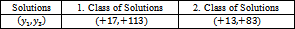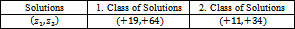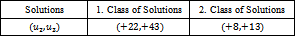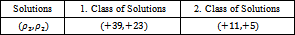## Tables index

#### Özen ÖZER

Turkish Journal of Analysis and Number Theory. 2019, 7(2), 50-55 doi:10.12691/tjant-7-2-4• Table 1. Solutions of 196x12-x32=5655 in integer• Table 2. Solutions of 49y12-y32=1392 in the set of integers• Table 3. Solutions of 49z12-z32=1305 in the set of integers• Table 4. Solutions of 4u22-u32=87 in the set of integers• Table 5. Solutions of 932 -2522=464 in the set of integers